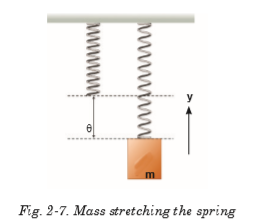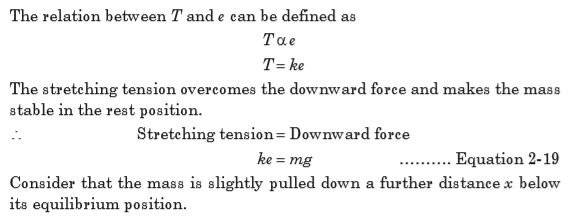## Topic outline

•• ### Unit 1: WAVE AND PARTICLE NATURE OF LIGHT

Unit 1: WAVE AND PARTICLE  NATURE OF LIGHT

Topic Area: LIGHT

Sub-Topic Area: Wave Particle Duality

Key unit competence: By the end of the unit, I should be able to analyze the nature of light.

Unit Objectives:

By the end of this unit learners will be able to;

◊ Explain the Planck’s quantum theory and apply it to other theories.

◊ Explain photoelectric effect and use it to derive and apply

Einstein’s photoelectric equation

◊ explain photoelectric effect and use it to derive and apply

Einstein’s photoelectric equation.

◊ Explain the wave theory  of light and state its limitations.

◊  Evaluate properties of light as a wave.

◊  Differentiate electron microscope and Compton Effect as applied in medicine.1.0 INTRODUCTION

Until the late 19th century physicists used to explain the phenomena in the physical world around them using theories such as mechanics, electromagnetism, thermodynamics and statistical physics that are known as classical theories.

At the turn of the 19th century, more and more experiments showed effects that could not be explained by these classical theories. This indicated a need for a new theory that we now know as quantum mechanics. Quantum mechanics is the system of laws which governs the behaviour of matter on the atomic scale. It is the most successful theory in the history of science, having withstood thousands of experimental tests without a single verifiable exception. So, the quantum mechanics is required to analyze the behaviour of photons, electrons and other particles that make up the universe.

This theory is the most useful in various studies especially for Radiography and Physiotherapy in Medicine, electrons and photons in Chemistry and Astronomy in Geography.

Opening question

Clearly observe the image shown on Fig.1-1, with kids playing on a slide with the help of their father Mr. John and answer the questions that follow.a)  Sarah is climbing the ladder. How do you think her potential energy    is changing?

b)  Comment on the potential energies of Jovia and Peter.

c) How is the change in the potential energy of Jovia as she slides down?

d) What do you think is Mr. John doing on the young kid? Give your comments.

Fig.1.2 below shows how light interacts with an electron. F and B are the terminals of the circuit (the wires of an external circuit).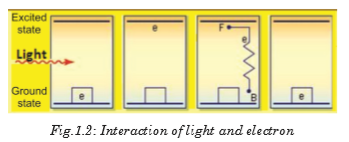The working mechanism of Fig.1.2 is used in solar cells and solar panels. Clearly analyse Fig.1.2 and compare it with the situation on Fig.1.1, take children as electrons at different points or positions, and make your comments.

1.1 PLANCK’S QUANTUM THEORY

The quantum theory arose out of the inability of the classical physics to explain the experimentally observed distribution of energy in the spectrum of a blackbody. When a blackbody is heated, it emits thermal radiations of different wavelengths or frequency. To explain these radiations, Max Planck put forward a theory known as Planck’s quantum theory. The theory is summarized in the following statements:

1. The matter is composed of a large number of oscillating particles. These oscillators have different frequencies.

2. The radiant energy which is emitted or absorbed by the blackbody is not continuous but discontinuous in the form of small discrete packets of energy and each such packet of energy is called a ‘quantum’. In case of light, the quantum of energy is called a ‘photon’.

3. The energy of each quantum is directly proportional to the frequency (f) of the radiation, i.e.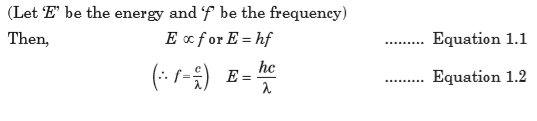whereas c is the speed of light, l is the wavelength and h is the Planck’s constant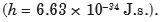4. The oscillator emits energy, when it moves from one quantized state to the other quantized state. The oscillator does not emit energy as long as it remains in one energy state. The total amount of energy emitted or absorbed by a body will be some whole number quanta. Hence,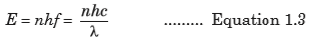where n is an integer.

According to the Planck’s theory, the exchange of energy between quantized states is not continuous but discrete. This quantized energy is in small packets of bundles. The bundle of energy or the packet of energy is called quantum (plural quanta).

EXAMPLE 1.1

The laser in a compact disc player. It uses light with a wavelength of
7.8 × 102 nm. Calculate the energy of a single photon of this light.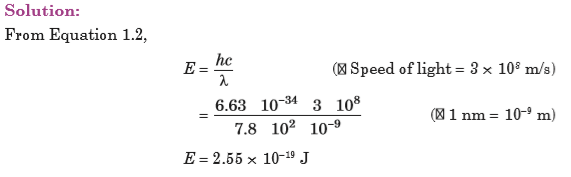EXAMPLE 1.2

What is the ratio between the energies of two radiations, one with a wavelength of 200 nm and the other with 600 nm?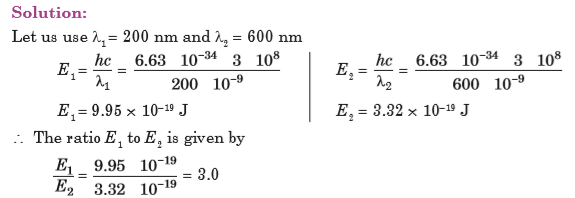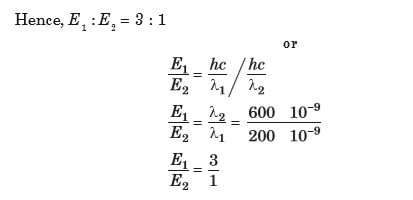EXERCISE 1.1

1. What is quantum mechanics?

2. What is Planck’s quantum theory?

3. Explain Planck’s hypothesis or what are the postulates of Planck’s quantum theory?

4. A laser emits light energy in short pulses with frequency 4.69 × 1014 Hz and deposits 1.3 × 10–2 J for each pulse.  How many quanta of energy does each pulse deposit?

1.2  PHOTON THEORY OF LIGHT AND PHOTOELECTRIC EFFECT

As proposed by Einstein, light is composed of photons, very small packets of energy. A photon has no mass and no charge. It is a carrier of electromagnetic energy and interacts with other discrete particles, e.g. electrons, atoms and molecules.

A beam of light is modelled as a stream of photons, each carrying a welldefined energy that is dependent upon the wavelength of the light. Einstein reasoned that to conserve energy, the light ought to be emitted in packets or quanta or photons and the energy of a given photon can be calculated by: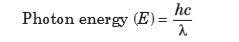where h is the Planck’s constant, c is the speed of light and l  is the wavelength.

Because all light ultimately comes from a radiating source, this idea suggests that light is transmitted as tiny particles, or photons as they are now called. The photon theory of light was also a radical departure from classical ideas. Einstein proposed a test of the quantum theory of light:

quantitative measurements on the photoelectric effect.The emission of electrons from the surface of metals, when light of a certain frequency is incident on it is known as photoelectric effect. In other words, it is the process of the removal of electrons from the surface of metal when the rays of special frequency fall on the surface of metal. As a result of the flow of these photoelectrons, the photoelectric current is produced.

Applications of photoelectric effect

There are numerous desirable applications based on photoelectric effect such as:

Automatic doors — The beam of light strikes the photocell, the photoelectric effect generates enough ejected electrons to produce a defectable electric current. When the light beam is blocked (by a person), the electric current is intruppted and the doors are signaled to open.

Solar panels — Photocells convert sunlight into electrical energy. Some other applications of photoelectric emission are: television, camera, tube light, activated counters, intrusion alarms, turn on street lights at dawn and safety lights.

1.3 WAVE THEORY OF MONOCHROMATIC LIGHT

Till the end of the 19th, century the predominant perception of wave propagation was moduled in some medium. Therefore, a medium called ether, was hypothesized for waves of light. The necessity of hypothesizing ether occured because it is different from any material medium known to us and it arises because light travels through empty space. For example, light coming from the sun and the stars as well as dense objects like glass, diamond can travel through empty space etc. Huygens published this theory in 1690, having compared the behaviour of light not with that of water waves but with that of sound. According to the Huygens’ principle:

• Light travels in the form of longitudinal waves which travel with uniform velocity in homogeneous medium.

• Different colours are due to the different wavelengths of light waves.

• We get the sensation of light when these waves enter our eyes.

• In order to explain the propagation of waves of light through vacuum. Huygens suggested the existence of a hypothetical medium called luminiferous ether, which is present in vacuum as well as in all material objects. Since ether couldn’t be detected, it was attributed properties like:

 It is continuous and is made up of elastic particles.

 It has zero density.

 It is perfectly transparent.

 It is present everywhere.

The Huygens’ principle of the wave theory of light states that:

“Every point on a wavefront may be considered a source of secondary spherical wavelets which spread out in the forward direction at the speed of light. The new wavefront is the tangential surface to all of these secondary wavelets.”In the wave theory assuming monochromatic light, the two important properties of a light wave are its intensity and frequency (or wavelength). When these two quantities are varied, the wave theory makes the following predictions:

1. if the light intensity is increased, the number of electrons ejected and their maximum kinetic energy should be increased because the higher intensity means greater electric field amplitude, and the greater electric field should eject the electrons with higher speed.

2. the frequency of the light should not affect the kinetic energy of the ejected electrons. Only the frequency of incident radiation affect the maximum kinetic energy.

There are, however, at least two problems with this idea and these led Newton and others to reject it:

(a) The secondary waves are propagated in the forward direction only, and

(b) They are assumed to destroy each other except when they form the new wavefront.

Newton wrote, ‘If light consists of undulations in an elastic medium, it should diverge in every direction from each new centre of disturbance. Like sound bend around all obstacles and obliterate all shadows.’ Newton did not know that in fact light behaves the same, but the effects are exceedingly small due to the very short wavelength of light.

(Huygens’ theory also failed to explain the rectilinear propagation of light.)

Definitions

A wave is any disturbance that results into the transfer of energy from one point to another point.

Primary source: The geometrical centre or axis of the actual source of light which is either a point or a line is called the primary source.

Wavelets: All points lying on small curved surfaces that receive light at the same time from the same source (primary or secondary) are called wavelets.

Secondary source: Any point on a wavelet, acts as the source of light for further propagation of light. It is called a secondary source.

Wavefront: The envelope of all wavelets in the same phase receives light from sources in the same phase at the same time is called a wavefront.

Wave normal: The normal at any point drawn outward on a wavefront is called the wave normal. Further propagation of light occurs along the wave normal. In isotropic media, the wave normal coincides with the ‘ray of light’.

Limitations of Huygens’ wave theory of light

• It could not explain the rectilinear propagation of light.

• It could not explain the phenomena of polarization of light such as Compton effect and Photoelectric effect.

• Michelson and Morley experiment concluded that there is no ether drag when the earth moves through it. This proves ether doesn’t exist. All the other attempts/ experiments to detect Luminiferous ether failed, which prove that it does not exist.

1.4 PROPERTIES OF A LIGHT WAVE

The properties of waves include the following:

The wavelength of a wave is defined as the distance over which the wave’s shape repeats.

It is the distance between the corresponding points on successive cycles, eg. the distance between two wave crests is known as wavelength of a sinusoidal wave. It is measured in units of length (metres, nanometres). The wavelength is usually represented by the symbol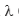(lambda).

A measurement of the wavelength is made by observing the wave in space at a single instant of time.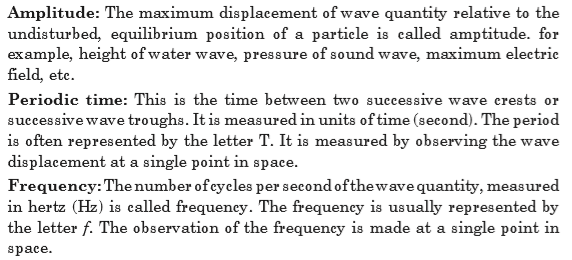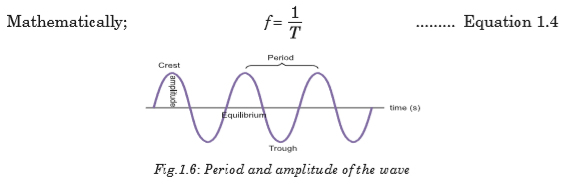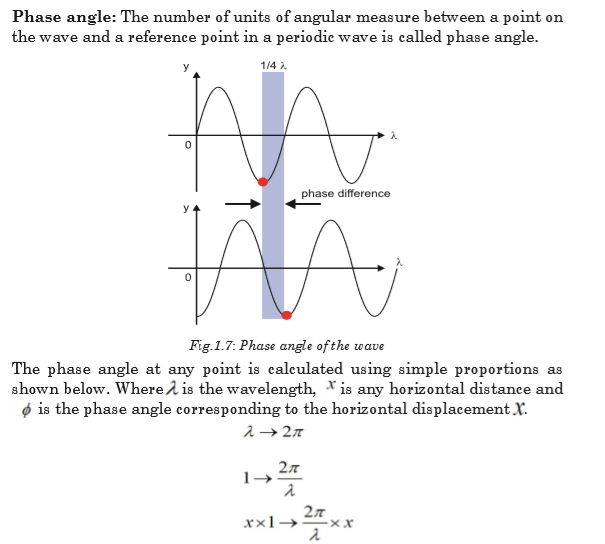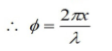1.5 BLACK BODY RADIATION

One of the earliest indications that classical physics was incomplete came from attempts to describe blackbody radiation. A blackbody is a theoretical object that absorbs 100% of the radiation that hits it and re-radiates energy which is the characteristic of this radiating system or body only. Therefore, it reflects no radiation and appears perfectly black.

The blackbody radiation is the emission of electromagnetic waves from the surface of an object. The distribution of blackbody radiation depends only the temperature of the object and is independent of the material.

This concept is idealized but can be very nearly realized in practice as illustrated in Fig. 1.9. The inner wall of the enclosure is black so that most of any radiation which enters through the small hole is absorbed on reaching the wall. The small amount of radiation which is reflected has very little chance of escaping through the hole before it is absorbed in a subsequent encounter with the wall.A blackbody radiator can be made by surrounding the enclosure of
Fig. 1.9(a) with a heating coil as shown on Fig.1.9(b). The radiation which is emitted by any section of the wall is involved in many reflections before it eventually emerges from the hole. Any section which is a poor emitter absorbs very little of the radiation which is incident on it, and those sections which are good emitters absorb most of the radiation which incident on them. This is the effect of mixing the radiations before they emerge and of making the temperature same at all points on the inner surface of the enclosure.1.6 ENERGY, MASS AND MOMENTUM OF A PHOTON

The famous Einstein equation of energy of the photon is E = mc2. In short, the equation describes how energy and mass are related with speed of light. To derive this equation, consider an X-ray photon of mass m hitting the surface of a metal and consider if a part of its energy is gained by a surface electron and is then emitted as shown in Fig. 1.10.The most important laws in dynamics are those that state the conservation of energy and the conservation of momentum. These two laws can be applied whenever we have a closed system; that is, a system that does not interact with its surroundings. They assert that for such systems and any process they may undergo:

Assume that;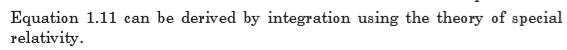EXERCISE 1.2

The mass of an electron or positron is 9.11 × 10–31 kg. The speed of light is 3.0 × 108 m/s.

1. Show that the rest energy of an electron is 8.2 × 10–14J.

2. Use the answer to question 1, to show that the rest energy of an electron is 0.51 MeV.

3. Write down the rest energy of a positron (antielectron).

4. An electron and a positron meet and annihilate one another. By how much does the rest energy decrease in total? Express the answer in MeV.

5. The annihilation of an electron and a positron at rest produces a pair of identical gamma ray photons travelling in opposite directions. Write down (in MeV) the energy you expect each photon to have.

6. A single photon passing near a nucleus can create an electron–positron pair. Their rest energy comes from the energy of the photon. Write down the smallest photon energy that can produce one such pair.

7. Cosmic rays can send high-energy photons through the atmosphere. What approximately is the maximum number of electron–positron pairs that a 10 GeV photon can create?

1.7 COMPTON EFFECT AND PHOTON INTERACTIONS

The Compton Effect concerns the inelastic scattering of X-rays by electrons. Scattering means dispersing in different directions and inelastic means that energy is lost by the scattered object in the process. The intensity of the scattered X-ray is measured as a function of the wavelength shift.

Photons are electromagnetic radiation with zero mass, zero charge, and a velocity that is always equal to  the speed of light. Because they are electrically neutral, they do not steadily lose energy via Coulombic interactions with atomic electrons, as charged particles do. Photons travel some considerable distance before undergoing a more “catastrophic” interaction leading to partial or total transfer of the photon energy to electron energy. These electrons will ultimately deposit their energy in the medium. Photons are far more penetrating than charged particles of similar energy. There are many types of photon interactions. We will only discuss those that are important in radiation therapy and/or diagnostic radiology.

1.7.1 Types of photon interactions

Coherent scattering

Coherent scattering is one of three forms of photon interaction which occurs when the energy of the X-ray or gamma photon is small in relation to the ionisation energy of the atom. It therefore occurs with low energy radiation. Upon interacting with the attenuating medium, the photon does not have enough energy to liberate the electron from its bound state (i.e. the photon energy is well below the binding energy of the electron), so no energy transfer occurs. The only change is a change of direction (scatter) of the photon, hence it is called ‘unmodified’ scatter. Coherent scattering is not a major interaction process encountered in radiography at the energies normally used. There are two types of coherent scattering: Thomson scattering and Rayleigh scattering.

• In Thomson scattering, only one electron of the atom is involved in the interaction.

• With Rayleigh scattering, all the electrons of the atom, sometimes called the electron cloud, are involved in a cooperative effort in the interaction with the photon.

Photoelectric effect

The following points make this phenomena clear:

1. The photon must have an energy equal to or greater than the binding energy of electron in the atom.

2. The incident photon must be completely absorbed by the electron.

3. The electron is then ejected from the atom.

4. The excess energy over the binding energy is given to the electron in the form of kinetic energy (which is the speed of the electron).

5. The hole left in the atom is filled by an outer shell electron or a free electron with the emission of characteristic radiation.

Compton interaction

In Compton interaction, the photon interacts with a ‘free’ or an outer shell electron. A portion of incident energy of the photon will be transferred to an electron in the form of kinetic energy. The incident photon, now called a scattered photon will be deflected in a new direction with less energy. Energy given to recoil electron is considered as the absorbed energy and the energy retained by the photon is considered scattered.

Pair Production

The photon interacts with the nuclear field of the atom, in such a way, that the photon transforms itself into an electron-positron pair. As the photon interacts with the strong electric field around the nucleus, it undergoes a change of state and is transformed into two particles (essentially creating matter from energy).

Photo disintegration

(Photo transmutation) It is a nuclear reaction in which the absorption of high energy electromagnetic radiation (a gamma-ray photon) causes the absorbing nucleus to change to another species by ejecting a subatomic particle, such as a proton, neutron, or alpha particle.1.8 WAVE PARTICLE DUALITY OF LIGHT

When one focusses on different types of phenomena such as interference, diffraction and polarisation, a wave picture of light can be built. This is because these phenomena are also found in properties of waves.

Experiments such as Young’s double slit experiment depend upon the diffraction of light waves and the constructive and destructive interferences show that light has wave-like properties.

The analysis of data in photoelectric experiment showed that the energy of ejected electrons was proportional to the frequency of illuminated light. This showed that whatever was knocking the electrons out had an energy proportional to frequency. The ejection energy of illumination showed that the interaction must be like that of a particle and gave all of its energy to the electron.

This fits well with Planck’s hypothesis that light in the blackbody experiment could exist only in discrete bundles of energy, so we conclude that light is a particle.

1.9 THE PRINCIPLE OF COMPLEMENTARITIES

The principle of complementarities refers to the effects such as wave particle duality in which different measurements made on the system reveal it to have either particle-like or wave-like properties. Both properties are necessary to gain the complete knowledge of the phenomena; they are complementary to each other; but at the same time, they also exclude each other.

Within the scope of classical physics, all characteristic properties of a given object can be ascertained by a single experimental arrangement, although in practice various arrangements are often convenient for the study of different aspects of the phenomena. In fact, data obtained in such a way simply supplement each other and can be combined into a consistent picture of the behaviour of the object under investigation. In quantum physics, however, evidence about atomic objects obtained by different experimental arrangements exhibits a novel kind of complementary relationship.

1.10 THE WAVE NATURE OF MATTER

Being fully aware of the pioneering work of Einstein on the photoelectric effect, de Broglie extended the notion of wave particle duality to matter. All matter can exhibit wave-like behaviour. For example, a beam of electron can be diffracted just like a beam of light or a water wave. The concept that matter behaves like a wave is also referred to de Broglie hypothesis. The de Broglie wavelength is the wavelength, l, associated with a massive particle and is related to its momentum p.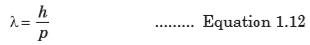With p being the particle’s momentum. The particles are diffracted by passing through an aperture in a similar manner as light waves. The wave properties of particles mean that when you confine it in a small space its momentum and kinetic energy must increase.

It is important to realize that the attribution of a wavelength to a massive particle implies that it should behave as a wave under some conditions. For example, it should be possible to verify this wave-like behavior when performing a diffraction experiment. Of course, there was no experimental evidence of any sort at the time to justify such an assertion.

To understand how a massive particle could exhibit wave-like properties, or how a wave could ‘behave as a particle, we must first review the notions of phase and group velocities.

1.11 ELECTRON MICROSCOPE

A microscope can be defined as an instrument that uses one or several lenses to form an enlarged (magnified) image. Microscopes can be classified according to the type of electromagnetic wave employed and whether this wave is transmitted or not through the specimen. The most common electron microscopes are Transmission Electron Microscope (TEM) and Scanning Electron Microscope (SEM).

1.11.1 Transmission electron microscope (TEM)

This is a microscopy technique whereby a beam of electrons is transmitted through an ultra thin specimen, interacting with the specimen as it passes through it. An image is formed from the electrons transmitted through the specimen, magnified and focused by an objective lens. It appears on an imaging screen, a fluorescent screen (in most TEMs), a monitor, or on a layer of photographic film. It can also be detected by a sensor such as a CCD camera. Theoretically, the maximum resolution that one can obtain with a light microscope has been limited by the wavelength of the photons that are being used to probe the sample and the numerical aperture of the system.

TEM consists of a cylindrical tube about 2 metres long. The tube contains vacuum where the specimen is located. This is because the molecules of gases, such as those in air, absorb electrons.

TEM works by emitting electrons from a cathode, then accelerating them through an anode, after which the electrons pass through an aperture into the vacuum tube.As it passes down through the tube the electron beam is controlled by electromagnetic lenses formed by coils around the tube (whose effect is moderated by adjusting the electricity flowing through the coils). These electromagnetic lenses direct the electron beam through the centre of the tube to a very thin specimen located part-way down the tube.

Some parts of the specimen might allow electrons to pass through them unaffected. Other regions within the specimen absorb some or all of the electrons that reach them. If any electrons continue from that part of the specimen further down the tube to the image formation plane with less energy. This happens because some of their energy has been absorbed by, or “passed to”, the part of the specimen that the electron(s) passed through.

TEM Applications

• TEMs provide topographical, morphological, compositional and crystalline information.

• It is useful in the study of crystals and metals, but also has industrial applications.

• TEMs can be used in semiconductor analysis and the manufacturing of computer and silicon chips.

• Tech giants use TEMs to identify flaws, fractures and damages to micro-sized objects; this data can help and fix problems and/or help to make a more durable efficient product.

• Colleges and universities can utilize TEMs for research and studies.

1.11.2 Scanning Electron Microscope (SEM)

The SEM is designed for direct study of the surfaces of solid objects. By scanning with an electron beam that has been generated and focussed by the operation of the microscope, an image is formed in the same way as a TV.The SEM allows a greater depth of focus than the optical microscope. For this reason, the SEM can produce an image that is a good representation of the three-dimensional sample.

The SEM uses electrons instead of light to form an image. A beam of electrons is produced at the top of the microscope by heating a metallic filament. The electron beam follows a vertical path through the column of the microscope. It makes its way through electromagnetic lenses which focus and direct the beam down towards the sample. Once it hits the sample, other electrons (backscattered or secondary) are ejected from the sample. Detectors collect the secondary or backscattered electrons, and convert them to a signal that is sent to a viewing screen similar to the one in an ordinary television, producing an image. To produce an image on the screen, the electron beam scans over the area to be magnified and transfers this image to the TV screen.

Applications of SEM

• Image morphology of samples (eg. view bulk material, coatings, sectioned material, foils, even grids prepared for transmission electron microscopy).

• Image composition and finding some bonding differences (through contrast and using backscattered electrons).

• Image molecular probes: metals and fluorescent probes.

• Undertake micro and nano lithography: remove material from samples; cut pieces out or remove progressive slices from samples (eg. using a focussed ion beam).

• Heat or cool samples while viewing them (it is generally done only in ESEM or during Cryo-scanning electron microscopy).

• Wet and dry samples while viewing them (only in an ESEM)

• View frozen material (in an SEM with a cryostage)

• Generate X-rays from samples for microanalysis (EDS; WDS) to determine chemical composition.

• Study optoelectronic behaviour of semiconductors using cathodoluminescence

• View/map grain orientation/crystallographic orientation and study related information like heterogeneity and microstrain in flat samples (Electron backscattered diffraction).

• Electron diffraction using electron backscattered diffraction. The geometry may be different from a transmission electron microscope but the physics of Bragg Diffraction is the same.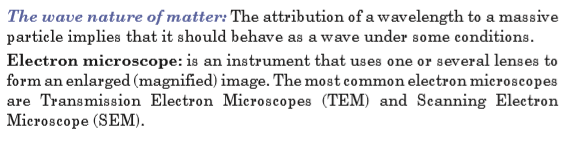URLs: 2Files: 2
• ### Unit 2: SIMPLE HARMONIC MOTION

Unit 2: SIMPLE HARMONIC MOTION

Topic Area: OSCILLATIONS AND WAVES

Sub-Topic Area: ENERGY CHANGES IN SIMPLE HARMONIC MOTION

Key unit competence: By the end of the unit I should be able to analyze energy changes in simple harmonic motion.

Unit Objectives:

By the end of this unit I will be able to;

◊ Determine the periodic time of an oscillating mass by practically and by calculation accurately.

◊ Derive and apply the equation of simple harmonic motion correctly

◊ Determine the periodic time of the simple pendulum correctly.2.0 INTRODUCTION

You are familiar with many examples of repeated motion in your daily life. If an object returns to its original position a number of times, we call its motion repetitive. Typical examples of repetitive motion of the human body are heartbeat and breathing. Many objects move in a repetitive way, such as a swing, a rocking chair and a clock pendulum. Probably the first understanding of repetitive motion grew out of the observations of motion of the sun and phases of the moon.

Strings undergoing repetitive motion are the physical basis of all string musical instruments. What are the common properties of these diverse examples of repetitive motion?

In this unit we will discuss the physical characteristics of repetitive motion and develop techniques that can be used to analyze this motion quantitatively.

Opening question

Clearly analyze the images of Fig. 2-1 given below and explain what you think will happen in each case when the mass is displaced.2.1 KINEMATICS OF SIMPLE HARMONIC MOTION

One common characteristic of the motions of the heartbeat, clock pendulum, violin string and the rotating phonograph turntable is that each motion has a well defined time interval for each complete cycle of its motion. Any motion that repeats itself with equal time intervals is called periodic motion. Its period is the time required for one cycle of the motion.Simple harmonic motion (SHM) is a type of motion where the restoring force is directly proportional to the displacement and acts in the direction opposite to that of displacement.

SHM is an oscillatory motion under a retarding force proportional to the amount of displacement from an equilibrium position. This means that Simple harmonic motion occurs when

• the force F acting on an object is directly proportional to the displacement x from a fixed point and is always towards this point.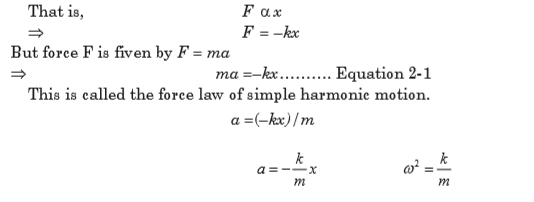• This means that acceleration is directly proportional to displacement from a fixed point and it is always directed towards this point.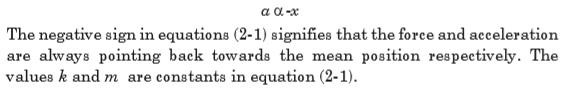Definition of terms

Time Period or Periodic Time T: It is the time taken for the particle to complete one oscillation, that is, the time taken for the particle to move from its starting position and return to its original position and is generally denoted by the symbol T.

Frequency f means how many oscillations occur in one second. Since the time period is the time taken for one oscillation, the frequency is expressed by; (f is frequency in one oscillation and T is the time period)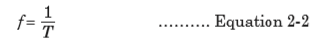The frequency is measured in s –1. This unit is known as the hertz (Hz) in honour of the physicist Heinrich Hertz.

Amplitude A is the maximum displacement of the particle from its resting position or mean position.Fig.2-3 shows the displacement-time graph of a periodic motion of a particle. From this graph, displacement can be represented as;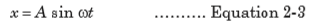Angular velocity (w): Angular velocity is the rate of change of angular displacement. It is measured in (rad/s). This is related to periodic time according to equation (2-4).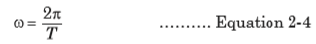Linear velocity (n): Linear velocity is the rate of change of linear displacement. It is measured in (m/s).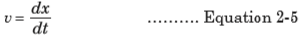Linear acceleration a of a particle is the rate of change of linear velocity of that particle with time. It is measured in m/s2.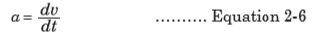2.2 EQUATION OF SIMPLE HARMONIC MOTION

The equation of simple harmonic motion is derived based on the conditions necessary for periodic motion to be simple harmonic.

Linear velocity can be related to displacement as shown below: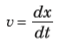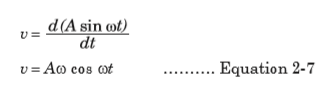But from trigonometric identities;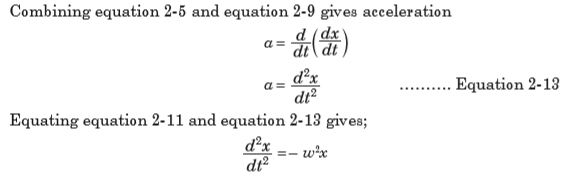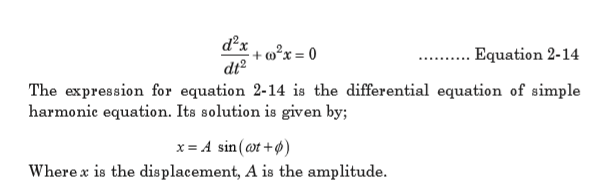EXAMPLE 2.1

A particle moving with SHM has velocities 4 cm/s and 3 cm/s at distances
3 cm and 4 cm respectively from equilibrium position. Find

(a) the amplitude of oscillation

(b) the period

(c) velocity of the particle as it passes through the equilibrium position.QUESTIONS

(i) Measure the gradient, m of your graph.

(j) Calculate the intercept c on the vertical axis.

(k) Calculate the constant a of the rule from c = log a.

(l) Calculate the period of a cantilever from T =  aLm

(m) Calculate the value of T from log T = m log L + log a  for value of
L = 70.0 cm.

(n) Compare and comment on the results in procedures (l) and (m).

EXAMPLE 2-3

The displacement of an object undergoing simple harmonic motion is given by the equation x(t) = 3.00 sin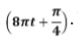. Where x is in meters, t is in seconds

and the argument of the sine function is in radians.

(a) What is the amplitude of motion?

(b) What is the frequency of oscillation?

(c) What are the position, velocity and acceleration of the object at t = 0?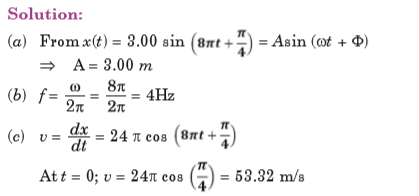2.3 SIMPLE HARMONIC OSCILLATORS

A simple harmonic oscillator is a physical system in which a particle oscillates above and below a mean position at one or more characteristic frequencies. Such systems often arise when a contrary force results from displacement from a force-neutral position and gets stronger in proportion to the amount of displacement. Below are some of the physical oscillators;

2.3.1 Simple Pendulum

A simple pendulum consists of a small bob of mass m suspended from a fixed support through a light, inextensible string of length L as shown on Fig.2-5. This system can stay in equilibrium if the string is vertical. This is called the mean position or the equilibrium position. If the particle is pulled aside and released, it oscillates in a circular arc with the center at the point of suspension ‘O’.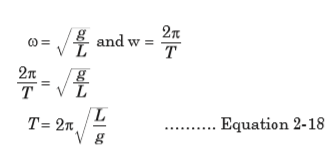Equation 2-18 represents the periodic time of a simple pendulum. Thus, the following are the factors affecting the periodic time of the simple pendulum;

• Length of string

• Angle from which pendulum is dropped

• Acceleration due to gravity

• Air resistance

EXAMPLE 2.4

A small piece of lead of mass 40 g is attached to the end of a light string of length 50 cm and it is allowed to hang freely. The lead is displaced to 0.5 cm above its rest position, and released.

(a) Calculate the period of the resulting motion, assuming it is simple harmonic.

(b) Calculate the maximum speed of the lead piece. (Take g = 9.81 ms–2)EXAMPLE 2.5

What happens to the period of a simple pendulum if the pendulum’s length is doubled? What happens to the period if the mass of the suspended bob is doubled?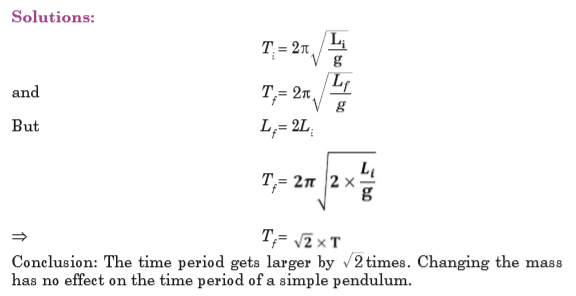2.3.2 Mass suspended from a Coiled Spring

The extension of the spiral spring which obeys Hook’s law is directly proportional to the extending tension. A mass m is attached to the end of the spring which exerts a downward tension mg on it and stretches it by e as shown in Fig.2-7 below;